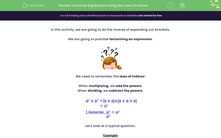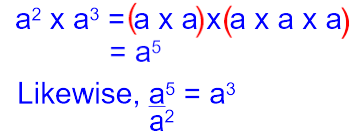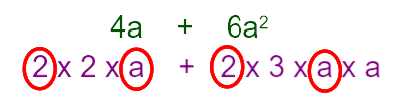# Factorise Expressions Using the Laws of Indices

In this worksheet, students will factorise expressions using the laws of indices.Key stage:  KS 3

Curriculum topic:   Algebra

Curriculum subtopic:   Simplify Algebraic Expressions to Maintain Equivalence

Difficulty level:#### Worksheet Overview

In this activity, we are going to do the inverse of expanding out brackets.

We are going to practise factorising an expression.We need to remember the laws of indices!

When multiplying, we add the powers.

When dividing, we subtract the powers.Let's look at a typical question.

Example

Factorise fully:

## 4a + 6a2

We look for the highest common factor of the terms:We can see from above the highest common factor is 2a, so that is what will go on the outside of the bracket.

We do 4a ÷ 2a =           and      6a2 ÷ 2a = 3a

## 4a + 6a2 = 2a(2 + 3a)

It's always a good idea to check that it works by expanding the newly factorised expression!

It really is easier than it looks, so let's get started!### What is EdPlace?

We're your National Curriculum aligned online education content provider helping each child succeed in English, maths and science from year 1 to GCSE. With an EdPlace account you’ll be able to track and measure progress, helping each child achieve their best. We build confidence and attainment by personalising each child’s learning at a level that suits them.

Get started